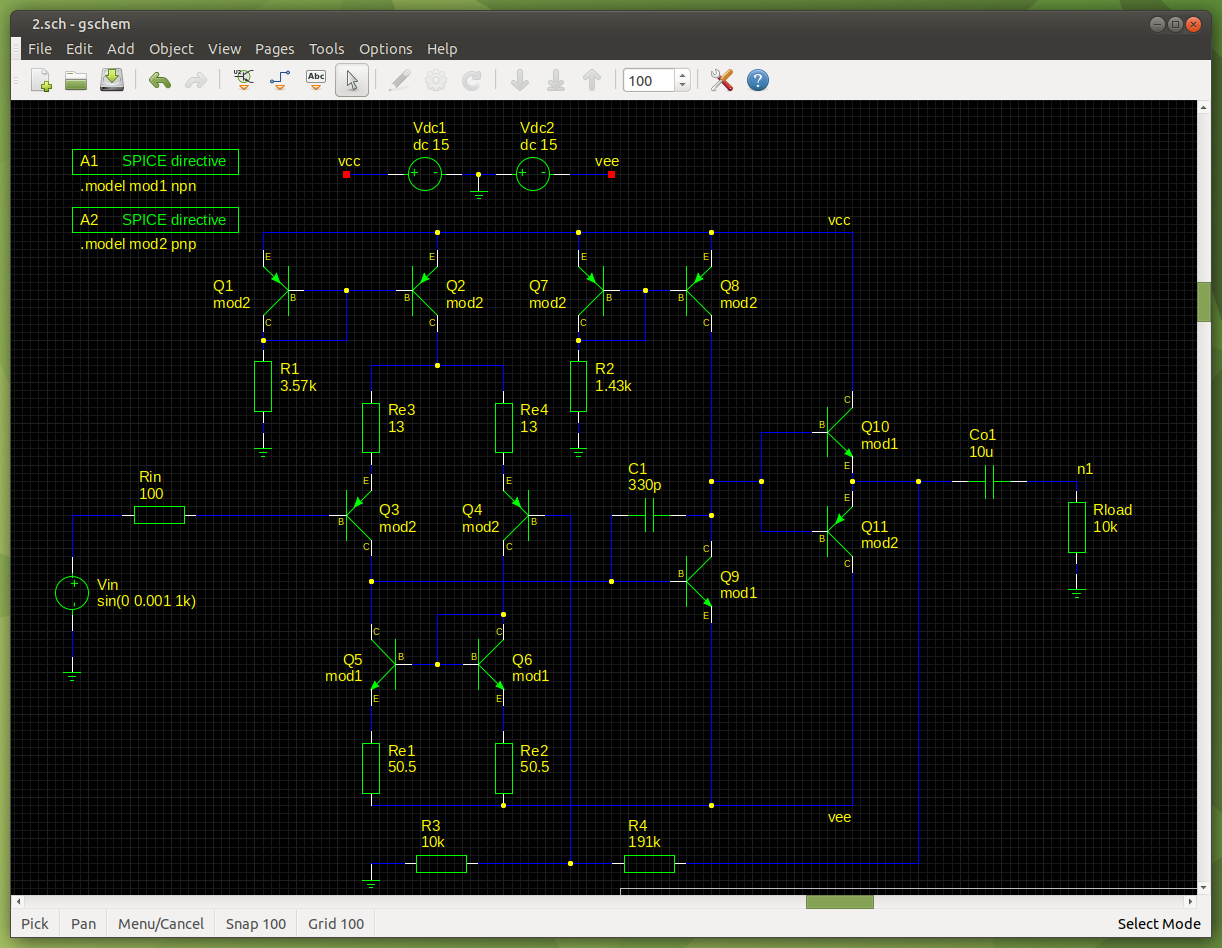# Input Linearity

To increase the linearity of the input stage, we reduce the non-linear intrinsic emitter resistance re and replace the missing part with an external linear resistance Re. To do this, we increase the collector current Ic, as re = 0.026/Ic at room temperature. At the moment the collector current is 1mA for each input transistor (26Ω re), let's double it, so there is 4mA tail current, 2mA flowing through each collector of the Long Tailed Pair. re is now 0.026/0.002 = 13Ω. We then replace the missing part of 13Ω with an external resistor (from the Audio Power Amplifier Design Handbook, Douglas Self, ISBN: 0 7506 56360). Note that as the emitter current has doubled, the voltage dropped across the current mirror emitter degeneration resistors has also doubled, so I halved the resistance to keep Vdegen the same.